# How to use the VLOOKUP function in Excel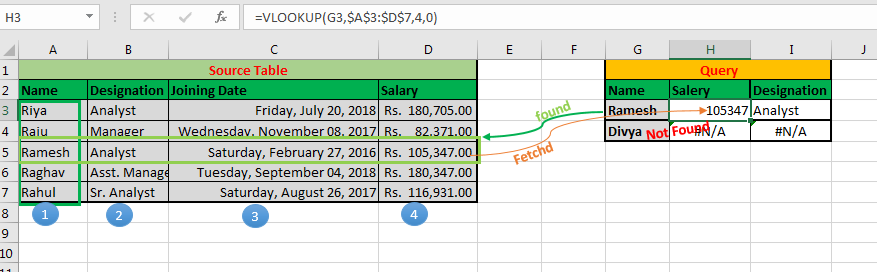Hi There! Imagine you have thousands of rows of data of employees in Excel, and your boss randomly asks you the salary and designation of Ramesh. Or worse than that, s/he gives you a list of hundred employees and asks you to send details of these employees. What would you do? You can’t find it manually. It is just not feasible and not smart at all. But you are smart, that’s why you are here. Let me introduce you to Excel’s VLOOKUP function.

### Why we Need VLOOKUP?

What is VLOOKUP? VLOOKUP stands for vertical lookup.

VLOOKUP just searches for the row of a given value in the first column of a table and returns asked column’s value in that row.

The Syntax of VLOOKUP:

=VLOOKUP(lookup_value, table_array, col_index_number, [range_lookup])

Lookup_value: The value by which you want to search in the first column of Table Array.

Table_array: The Table in which you want to look up/search

col_index_number: The column number in Table Array from which you want to fetch results.

[range_lookup]: FALSE if you want to search for exact value, TRUE if you want an approximate match.

Ok, enough of the theory. Let's jump into an Excel VLOOKUP example.

## VLOOKUP Example 1.

In an Excel sheet, you have this data from employees. In the first column (Column A), you have the name of employees. Next, you have their designation and so on as shown in the image below.

To make it easy, I have taken a small table.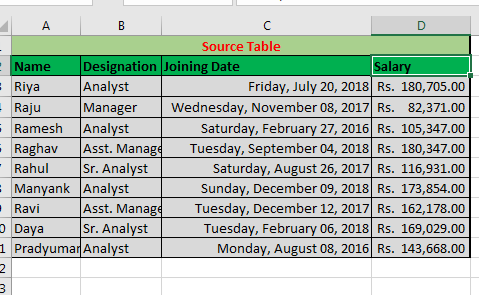Now your bossy boss has sent you this list and asked for details in blank columns.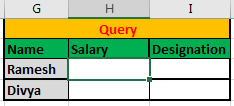You need to tell Ramesh and Divya’s Salaries and Designations. How would you do that?

To do this you can search manually Or… Or you can use the Excel VLOOKUP function.

Just write this VLOOKUP formula in Cell H2 and copy it in below cell:

=VLOOKUP(G3,\$A\$3:\$D\$11,4,FALSE)

You will get the results as shown below.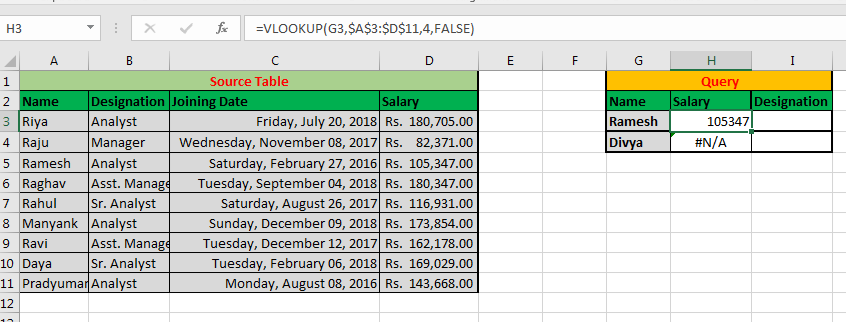Explanation:
Here we told excel to lookup for Ramesh (G3) in the first column (A) of table \$A\$3:\$D\$11.

If the given value is found then return the value in the 4th (4) column on that table.

By giving it a FALSE in range_lookup, you are telling VLOOKUP to find the exact match (No ifs n buts).

Here Ramesh is found in first column A and his salary is returned as 105347.

Similarly, VLOOKUP looks for Divya in a column and returns #N/A error since Divya’s record is not there in your table.

Do you see the other use of VLOOKUP here? You can use VLOOKUP function to know if a value exists in a list or not. You can also match two lists and know what values are common in them.

Pro Tip: always lock the reference of Table_array when using VLOOKUP in Excel. Otherwise, your table_array will change when you copy your formula in other cells.

It is by default when you VLOOKUP from another sheet or workbook but in the same sheet, you need to do it manually.

Can you write the VLOOKUP formula to get Ramesh’s designation? The comments section is all yours.

In the above example, we saw a VLOOKUP Example where we wanted an exact match. But what if we want to know the approximate match?

When do you use the approximate match in VLOOKUP as Range_lookup?

Let’s understand it with another VLOOKUP example.

### Example 2: Use of Approximate Match in VLOOKUP

You correctly answered your boss's query using VLOOKUP previously. Now your “dear” boss wants to know the nearest salary to 150000 (only less than).
To answer this, we will again use VLOOKUP but this time with an approximate match.

Important note: To find an approximate match, you need your data to be sorted. Here, I have sorted data in ascending order by salary.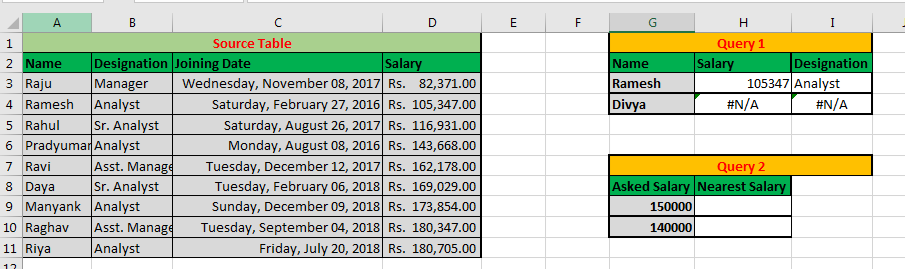In cell H2 write this formula and drag it to the cell below:

=VLOOKUP(G9,\$D\$3:\$D\$11,1,1)

Note: 1 is interpreted as TRUE and 0 as FALSE in Excel.

Ultimately you will have a result that looks like this: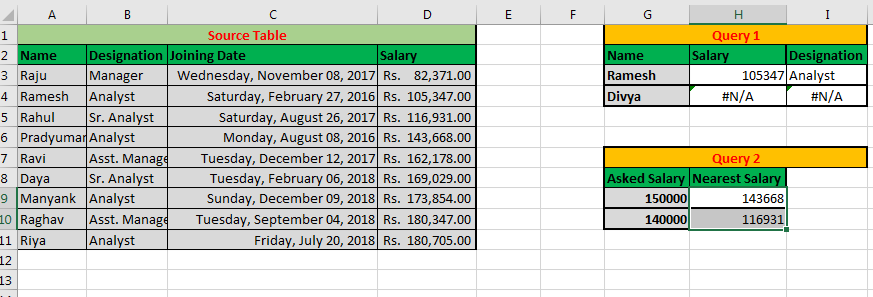Explanation.
Our lookup Value is 150000 (G9) and we want the nearest salary to this.
Our range is \$D\$3:\$D\$11 since we want to return the value from the same column. Therefore, col_index_num is also 1. And since we want an approximate match we supplied range_lookup as 1 (TRUE).

For an approximate match, your data needs to be sorted. Otherwise Excel VLOOKUP will serve as an incorrect result.

Important Notes:

• VLOOKUP always works Left to Right. You can’t fetch a value from the right of the first column.
• Column Numbering Starts from the select range. For example, if you supply table array D4:F10. Then the column count will start with D, not from the actual start of the table.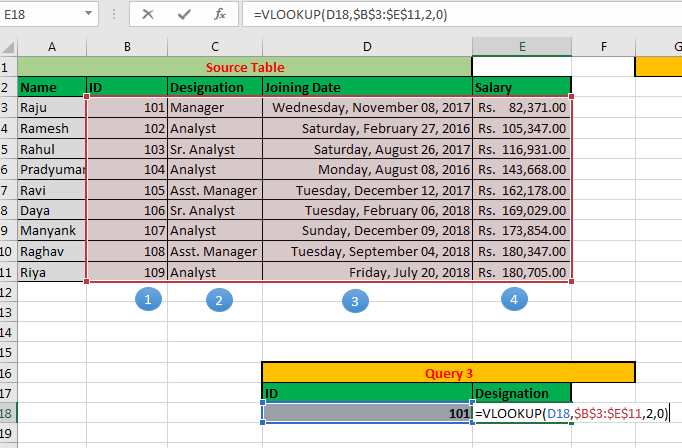• Always lock-in reference to Table using \$sign to avoid error while copying the V lookup formula in Microsoft Excel 2016.
• You can use wild card character (*) in lookup Value when you only remember some part of lookup Value.
• Set Range_Lookup to FALSE (0) to get an exact match to your value.
• Set Range_Lookup to TRUE (1) to get the nearest to less than to the given value.
• Always sort data in ascending order for an approximate match.

Now you can answer your boss's query in seconds. You can compare two lists easily. You can do a bulk search using VLOOKUP in Excel 2016, 2013, 2010 and in older versions of Excel in seconds.

Related Articles

Vlookup Top 5 Values with Duplicate Values Using INDEX-MATCH in Excel

VLOOKUP Multiple Values

VLOOKUP with Dynamic Col Index

Partial match with VLOOKUP function

Use VLOOKUP from Two or More Lookup Tables

Vlookup by Date in Excel

Using a VLOOKUP Formula to Check If a Value Exists

Popular Articles

How to VLOOKUP from Different Excel Sheet

VLOOKUP with numbers and text

IF, ISNA and VLOOKUP function

ISNA and VLOOKUP function

IFERROR and VLOOKUP function

Terms and Conditions of use

The applications/code on this site are distributed as is and without warranties or liability. In no event shall the owner of the copyrights, or the authors of the applications/code be liable for any loss of profit, any problems or any damage resulting from the use or evaluation of the applications/code.